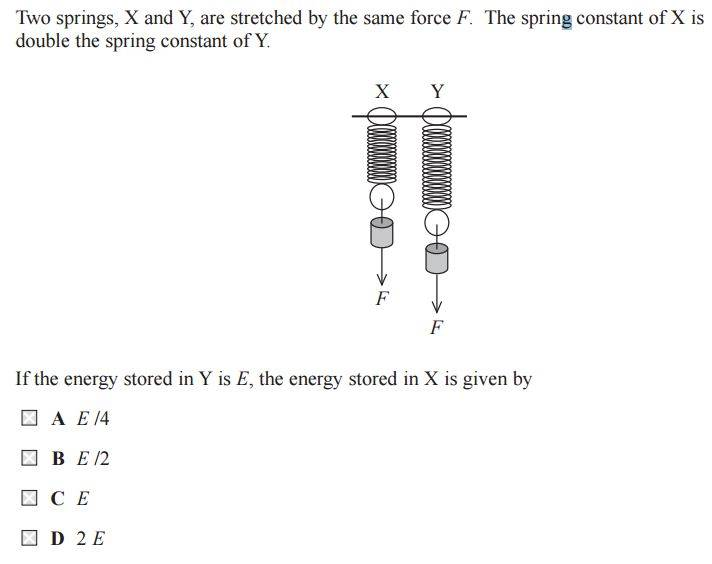# Spring constant and energy

## Homework Statement## The Attempt at a Solution

EPE is (0.5)kx^2

Let Y have spring constant of K then X has spring constant of 2K

So EPE of Y is (0.50kx^2 which is E

So EPE of X must be (0.5)2kx^2 which is kx^2 which is 2E? But correct answer is E/2??

gneill
Mentor
It looks like you've assumed that x is the same for both springs. Is that going to be the case?

It looks like you've assumed that x is the same for both springs. Is that going to be the case?

Ah, in that case if X has a stiffer spring, 2K, then its extension (x) will only be half, so we'd have (0.5)(2K)(0.5x) so the energy stored is the same for both??

gneill
Mentor
Ah, in that case if X has a stiffer spring, 2K, then its extension (x) will only be half, so we'd have (0.5)(2K)(0.5x) so the energy stored is the same for both??
Check the expression you've just used for the energy. Did you forget something?

•ravsterphysics
Check the expression you've just used for the energy. Did you forget something?

Argh such a silly mistake! Thanks for your help : )